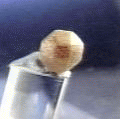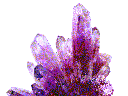Diamond
Tetragonal | Hexagonal | Trigonal | Orthorhombic | Monoclinic | Triclinic | Amorphous

# THE ISOMETRIC SYSTEM

SOME ISOMETRIC
MINERALS

SPINEL

LAZURITE

ANALCIME

GALENA

GOLD

FLUORITE

ALMANDINE

HALITE

COBALTITE

DIAMOND

TETRAHEDRITE

BIXBYITE

The isometric system is the most symmetrical system possible in three dimensional space. It is composed of three crystallographic axes of equal length and at right angles to each other. It differs from the other systems in many ways. Isometric crystals do not polarize light as it passes through them, making them more similar, in this case, to amorphous substances such as glass. In addition, the crystallographic axes, while they do serve as some of the symmetry elements, are not the principle axes of symmetry, as is true in the other systems. All the isometric crystal forms are closed forms and exclusive to this system. No isometric crystals will have faces that belong to pyramids, prisms, rhombohedrons, scalahedrons, domes, sphenoids, pinacoids or pedions.

The word, isometric, means "same measure", an allusion to the equal three dimensional structure. "Cubic System" is a synonym for the isometric system and is due to the cubic nature of the crystallographic structure as well as the most common isometric form, the cube.

The most symmetrical class of all is the Hexoctahedral Class. The three identical crystallographic axes serve as four fold rotational axes with a square outline when viewed down each axis. However the principle and defining axes for the isometric system are the 4 three fold axes. They dissect the three crystallographic axes and are essentially the 4 diagonal lines through a cube. If you picture a transparent cube, then draw lines from each corner, through the middle to the other corner, then you will end up with 4 lines that represent the 4 three fold axes. The three fold rotational axes can be see on a cube as the axes through the three top faces and the three bottom faces, when the cube is held from corner to opposite corner.

The octahedron shows the three fold rotations better than the cube with its triangular faces that are perpendicular to the three fold axes. The four fold rotations though are not as easy to see on the octahedron, but a square cross-section can be seen when viewed down the points of the octahedron. The other forms of the hexoctahedral class all demonstrate these symmetry elements including the 6 two fold axes and 9 mirror planes.

The Hextetrahedral Class lacks the 3 simple four fold rotational axes because they are four fold rotoinversion axes. A four fold rotoinversion axis takes a face, rotates it 90 degrees (one fourth of a rotation) and then inverts it (up to down & right to left) through the crystal to the other side. Then it rotates it again 90 degrees and inverts it again through the crystal. Another rotoinversion operation and finally another (four in all) and the face is back, exactly where it started. The crystal then looks as if it has only two fold axes instead of the four fold axes that it actually has. The hextetrahedral class produces a very symmetrical tetrahedron and derivatives of this form. The three fold axes are very easy to see in this class as they run through the triangular bases and out the three faced pyramidal top. Of course there are no bases or pyramids in the isometrmic system these terms are used only as a visual guide to the shape of the tetrahedron.

The Gyroidal Class has all axes of the hexoctahedral class but none of the mirror planes. This class produces a unique form called the gyroid. It has 24 faces that are in the shape of distorted pentagons. The lack of mirror planes is clearly seen on the gyroid. This class like the other classes can have forms that appear to be of higher symmetry such as the cube. But etchings on crystal faces or other factors such as striations can be used to reveal the true symmetry of the crystal.

The Diploidal Class lacks any four fold rotational axes or any four fold rotoinversion axes. Remember, the isometric system doesn't require four fold rotational axes. There is the required 4 three fold axes as well as 3 two fold axes (parallel to the crystallographic axes), three mirrors and a center. The class contains a few rare minerals and one very common, well known and usually well crystallized mineral, that being pyrite. Pyrite lends its name to the classic form that comes from this class and the form that pyrite is famous for, the pyritohedron. Although pyrite can form clean perfect cubes, it usually forms cubes that are striated with fine grooves that run across the cube faces. The striations are the result of the pyrite crystal trying to express its other form, the pyritohedron. A careful examination of the pyritohedron and a cube show that the cube faces and six of the edges on the pyritohedron are aligned crystallographically. Therefore the edges imprint on the cube face as striations. These striations ruin the cube's normal four fold axis that would have entered the crystal in the middle of the cube face and this proves that pyrite belongs to the Diploidal Class.

The lowest symmetry class in the isometric system is the Tetartoidal Class. It lacks any mirror planes or a center, but has the required 4 three fold axes in addition to 3 two fold axes that lie on the crystallographic axes. The tetartoid is a unique form and is a 12 sided form that peripherally resembles the shape of a tetrahedron.

## The Hexoctahedral Symmetry ClassFluorite

^

## The Hextetrahedral Symmetry ClassSphalerite

• Class: 31st
• Symmetry: Bar 4 3 m
• Symmetry Elements: There are 4 three fold axes, 3 four fold rotoinversion axes (they appear as two fold axes) and 6 mirror planes.
• Crystallographic Axes: Three equal axes termed a1, a2 and a3.
• Angles: All three angles = 90 degrees.
• Common Forms: The tetrahedron, tristetrahedron, deltoidal dodecahedron and hextetrahedron, and more rarely the cube, rhombic dodecahedron and tetrahexahedron.
• Most Common Minerals: Sodalite, sphalerite, domeykite, hauyne, zunyite, helvite, metacinnabar, hawleyite, lazurite, rhodizite, tetrahedriteand tennantite along with several other rare minerals.
^

## The Gyroidal Symmetry ClassCuprite

• Class: 30th
• Symmetry: 4 3 2
• Symmetry Elements: There are 3 four fold axes, 4 three fold axes and 6 two fold axes.
• Crystallographic Axes: Three equal axes termed a1, a2 and a3.
• Angles: All three angles = 90 degrees.
• Of Note: Crystals can be left or right handed.
• Common Forms: The unique gyroid form as well as the cube, octahedron, dodecahedron and trapezohedron, more rarely the trisoctahedron and tetrahexahedron.
• Most Common Minerals: Lawsonite, petzite, fischesserite, sakhaite and yeelimite. The minerals cuprite, voltaite and sal ammoniac are in dispute as to belonging to this class, but sometimes show crystal forms consistent with this class.
^

## The Diploidal Symmetry ClassPyrite

• Class: 29th
• Symmetry: 2/m bar 3
• Symmetry Elements: There are 4 three fold axes, 3 two fold axes, 3 mirror planes and a center.
• Crystallographic Axes: Three equal axes termed a1, a2 and a3.
• Angles: All three angles = 90 degrees.
• Common Forms: The unique diploid and pyritohedron as well as the cube, octahedron, rhombic dodecahedron, trapezohedron and more rarely the trisoctahedron.
• Most Common Minerals: Pyrite, cobaltite, cliffordite, hauerite, ullmannite, penroseite, tychite, laurite, skutterudite, bixbyite and sperrylite.
^

## The Tetartoidal Symmetry Class• Class: 28th
• Symmetry: 2 3
• Symmetry Elements: There are 4 three fold axes and 3 two fold axes.
• Crystallographic Axes: Three equal axes termed a1, a2 and a3.
• Angles: All three angles = 90 degrees.
• Of Note: Crystals can be left or right handed, enantiomorphic.
• Common Forms: The unique tetartoid as well as the pyritohedron, cube, deltoidal dodecahedron, pentagonal dodecahedron, rhombic dodecahedronand the tetrahedron.
• Most Common Minerals Known to this Class: Include very rare minerals such as Changchengite, corderoite, gersdorffite, langbeinite, maghemite, michenerite, pharmacosiderite, ullmannite (higher ordered) and a few other very rare minerals.
^

Tetragonal | Hexagonal | Trigonal | Orthorhombic | Monoclinic | Triclinic | AmorphousAmethyst Galleries'Mineral Gallery MINERALS Groupings Birthstones Gemstones By Name Framed Unframed By Class Carbonates Elements Halides Oxides Phosphates Silicates Sulfates Sulfides Fluorescent Minerals Properties Rocks Search Books